x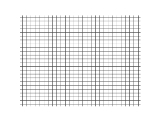Longitudinal waveEncyclopedia
Longitudinal waves, as known as "l-waves", are waves that have the same direction of vibration as their direction of travel, which means that the movement of the medium is in the same direction as or the opposite direction to the motion of the wave. Mechanical longitudinal waves have been also referred to as compressional waves or compression waves.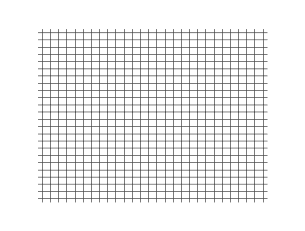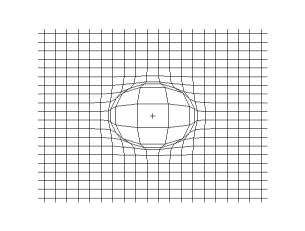## Non-electromagnetic

Longitudinal waves include sound waves (alternation in pressure, particle displacement, or particle velocity propagated in an elastic material) and seismic P-wave
P-wave
P-waves are a type of elastic wave, also called seismic waves, that can travel through gases , solids and liquids, including the Earth. P-waves are produced by earthquakes and recorded by seismographs...

s (created by earthquakes and explosions).

### Sound waves

In the case of longitudinal harmonic sound waves, the frequency
Frequency
Frequency is the number of occurrences of a repeating event per unit time. It is also referred to as temporal frequency.The period is the duration of one cycle in a repeating event, so the period is the reciprocal of the frequency...

and wavelength
Wavelength
In physics, the wavelength of a sinusoidal wave is the spatial period of the wave—the distance over which the wave's shape repeats.It is usually determined by considering the distance between consecutive corresponding points of the same phase, such as crests, troughs, or zero crossings, and is a...

can be described with the formula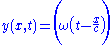where:
• y is the displacement of the point on the traveling sound wave;
• x is the distance the point has traveled from the wave's source;
• t is the time elapsed;
• y0 is the amplitude of the oscillations,
• c is the speed of the wave; and
• ω is the angular frequency of the wave.

The quantity x/c is the time that the wave takes to travel the distance x.

The ordinary frequency (f) of the wave is given by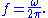For sound waves, the amplitude of the wave is the difference between the pressure of the undisturbed air and the maximum pressure caused by the wave.

Sound's propagation speed depends on the type, temperature and pressure of the medium through which it propagates.

### Pressure waves

In an elastic medium with rigidity, a harmonic pressure wave oscillation has the form,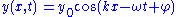where:
• y0 is the amplitude of displacement,
• k is the wavenumber
Wavenumber
In the physical sciences, the wavenumber is a property of a wave, its spatial frequency, that is proportional to the reciprocal of the wavelength. It is also the magnitude of the wave vector...

,
• x is distance along the axis of propagation,
• ω is angular frequency,
• t is time, and
• φ is phase difference.

The force acting to return the medium to its original position is provided by the medium's bulk modulus
Bulk modulus
The bulk modulus of a substance measures the substance's resistance to uniform compression. It is defined as the pressure increase needed to decrease the volume by a factor of 1/e...

.

## Electromagnetic

Maxwell's equations
Maxwell's equations
Maxwell's equations are a set of partial differential equations that, together with the Lorentz force law, form the foundation of classical electrodynamics, classical optics, and electric circuits. These fields in turn underlie modern electrical and communications technologies.Maxwell's equations...

lead to the prediction of electromagnetic waves in a vacuum, which are transverse (in that the electric fields and magnetic fields vary perpendicularly to the direction of propagation). However, waves can exist in plasma or confined spaces. These are called plasma waves and can be longitudinal, transverse, or a mixture of both. Plasma waves can also occur in force-free magnetic fields.

In the early development of electromagnetism there was some suggesting that longitudinal electromagnetic waves existed in a vacuum. After Heaviside's attempts to generalize Maxwell's equations, Heaviside came to the conclusion that electromagnetic waves were not to be found as longitudinal waves in "free space" or homogeneous media. But it should be stated that
Maxwell's equations do lead to the appearance of longitudinal waves under some circumstances in either plasma waves or guided waves. Basically distinct from the "free-space" waves, such as those studied by Hertz in his UHF experiments, are Zenneck waves. The longitudinal mode of a resonant cavity is a particular standing wave pattern formed by waves confined in a cavity. The longitudinal modes correspond to the wavelengths of the wave which are reinforced by constructive interference after many reflections from the cavity's reflecting surfaces. Recently, Haifeng Wang et al. proposed a method that can generate a longitudinal electromagnetic (light) wave in free space, and this wave can propagate without divergence for a few wavelengths.

• Varadan, V. K., and Vasundara V. Varadan, "Elastic wave scattering and propagation". Attenuation due to scattering of ultrasonic compressional waves in granular media - A.J. Devaney, H. Levine, and T. Plona. Ann Arbor, Mich., Ann Arbor Science, 1982.
• Schaaf, John van der, Jaap C. Schouten, and Cor M. van den Bleek, "Experimental Observation of Pressure Waves in Gas-Solids Fluidized Beds". American Institute of Chemical Engineers. New York, N.Y., 1997.
• Krishan, S, and A A Selim, "Generation of transverse waves by non-linear wave-wave interaction". Department of Physics, University of Alberta, Edmonton, Canada.
• Barrow, W. L., "Transmission of electromagnetic waves in hollow tubes of metal", Proc. IRE, vol. 24, pp. 1298–1398, October 1936.
• Russell, Dan, "Longitudinal and Transverse Wave Motion". Acoustics Animations, Kettering University Applied Physics.
• Longitudinal Waves, with animations "The Physics Classroom"